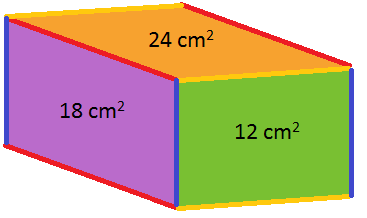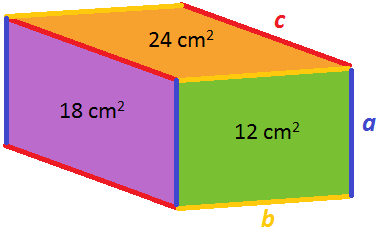#### You may also like### Hallway Borders

What are the possible dimensions of a rectangular hallway if the number of tiles around the perimeter is exactly half the total number of tiles?### Square Pegs

Which is a better fit, a square peg in a round hole or a round peg in a square hole?### Boxed In

A box has faces with areas 3, 12 and 25 square centimetres. What is the volume of the box?

# Cuboid Faces

##### Age 11 to 14 ShortChallenge Level
Working out the lengths of the edgesFrom this diagram, we can see that:
• the length of the yellow edge multiplied by the length of the blue edge must be 12
• the length of the red edge multiplied by the length of the blue edge must be 18
• the length of the yellow edge multiplied by the length of the red edge must be 24.
We can start by thinking of two numbers that multiply to get 12, and then choose the right number to make the purple face have an area of 18 square centimetres. Then we can check whether those numbers give us the correct area for the orange face.

If the yellow edge is 6 cm long and the blue edge is 2 cm long, then the red edge must be 9 cm long, because 2$\times$9 = 18. However, then the orange face would have area 6$\times$9 = 54 square centimetres, which is not right.
If the yellow edge is 2 cm long and the blue edge is 6 cm long, then the red edge must be 3 cm long, because 6$\times$3 = 18. However, then the orange face would have area 2$\times$3 = 6 square centimetres, which is not right.
If the yellow edge is 4 cm long and the blue edge is 3 cm long, then the red edge must be 6 cm long, because 3$\times$6 = 18. Then the orange face would have area 4$\times$6 = 24 square centimetres, which is right.
So the edges of the cuboid are 3 cm, 4 cm and 6 cm long, which means the volume of the cuboid is 3$\times$4$\times$6 = 72 cm$^3$.

Using algebra to find the lengths of the edgesIf we call the lengths of the edges $a$, $b$ and $c$, then $ab=12$, $ac=18$ and $bc=24$.

From here, we can use a trial approach to work out $a$, $b$ and $c$ similar to the method described above, or we can solve these equations, as shown below.

Since $ab=12$, we can say that $a=\dfrac{12}{b}$.

Substituting into $ac=18$, we get $\dfrac{12}{b}\times c=18$. Rearranging, $12c=18b$, so $c=\dfrac{18b}{12}=\dfrac{3b}{2}$.

Substituting into $bc=24$ now gives $b\times\dfrac{3b}{2}=24$, so $\dfrac{3b^{2}}{2}=24$, so $b^{2}=16$, so $b=4$.

So $a=\dfrac{12}{4}=3$ and $c=\dfrac{3\times4}{2}=6$.

So the volume, given by $abc$, is equal to $3\times4\times6=72$ cm$^3$.

Using algebra to find the volume directly
As in the picture above, we can call the lengths of the edges $a$, $b$ and $c$. So $ab=12$, $ac=18$ and $bc=24$.

We want the volume of the cuboid, $V=abc$.

Notice that $ab\times ac\times bc=a^2b^2c^2=(abc)^2=V^2$.
So $12\times18\times24=V^2$.
So $V=\sqrt{12\times18\times24}=72$.
So the volume is $72$ cm$^3$.
You can find more short problems, arranged by curriculum topic, in our short problems collection.